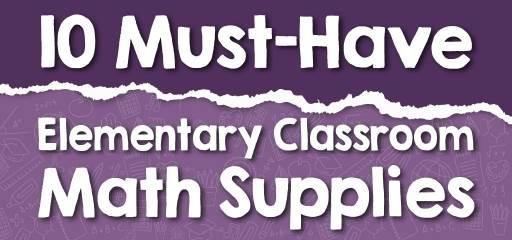# 10 Must-Have Elementary Classroom Math SuppliesMath can be a very boring lesson for elementary school students. In this case, what can be done to change this view in students? One way to make math lessons engaging for elementary students is to use math supplies in the classroom. So, in this article, we are going to introduce you to 10 elementary classroom math supplies that you can count on.

## 1- Math Posters

Math posters are great tools for showing a summary of important information. This is a very important feature that makes necessary things such as formulas and symbols visible to elementary students to make them easier to remember. Such posters also help students to make a better connection with math problems.

## 2- 1-100 Charts

These charts are great tools for teaching numbers to elementary students. These charts can be easily hung on the classroom wall or bulletin boards and used for classroom instruction. Using this method, learning numbers can be made easy and attractive for elementary students. These charts can even be used in classroom activities if you ask your students to arrange the numbers themselves.

## 3- Balance

balance is suitable for teaching many mathematical concepts. With this mathematical tool, you can show the early algebraic equations hey balance equivalent numbers on both sides. It can also be used to show student’s operations and equivalent numbers. It is a great tool for learning number relationships and concepts such as weight.

## 4- Magnetic Ten-Frame Set

You can use the Magnetic Ten-Frame Set to make learning math more fun for your elementary students. This product is suitable for teaching basic mathematical concepts such as counting, number patterns, operations. Due to their magnetic features, they are suitable for both whiteboard and student desks. This supply can also be useful for elementary students’ math activities at home.

## 5- Teaching Clock

There are tools like the teaching clock for teaching time to elementary students. Learning how time works is a necessity for everyone, so you can make it easier with the teaching clock. This type of clock has a hidden gear mechanism that automatically advances the hour hand when the minute hand is manipulated manually. This feature helps the student become familiar with the use of the clock in a step-by-step manner.

## 6- Geometric Solids

Familiarity with geometric shapes visually can be more appealing to elementary students. As a result, you can use supplies that are specifically designed for this purpose. Using geometric solids, sides, angles, and surfaces can be easily shown to students so that they can understand how to achieve geometric formulas in the future.

## 7- Write & Wipe Fact Family Boards

Using this attractive product, students can discover the relationship between the numbers. Working with such a tool in the math class also develops the student’s higher-level algebraic skills such as equation building, commutative property, and problem-solving. With the help of Write & Wipe Fact Family Boards, skills such as addition, subtraction, multiplication, and division can be taught to students simply.

## 8- Number Line Sets

Another interesting supply for teaching numbers and their use is number line sets. These sets are used to teach students the subject of integers and absolute values. For students who are interested in hands-on learning, using this tool in the classroom can help a lot in learning number recognition, sequencing, counting, and more.

## 9- Base Ten Blocks

As a teacher, you can use Base Ten Blocks to teach many basic concepts. These blocks are useful for group teaching in math class and suitable for grades 1 and up. With this product, the student can focus more on concepts such as addition, subtraction, multiplication, and division and learn them better.

## 10- Dry Erase XY Axis Graph Lap Boards

These boards have an XY axis that makes them suitable for math practice. On the other side of these boards is a blank writing surface that students can use both to do math exercises and to practice other lessons at home and in the classroom. Using these boards facilitates interactive learning for students. You can use this tool to increase students’ classroom activity.

This list is just a few of our choices for elementary school teachers to use. With a little creativity and spending time, you can find many other supplies that increase the interactive effective learning and the ability of your elementary students to learn math content.

### What people say about "10 Must-Have Elementary Classroom Math Supplies - Effortless Math: We Help Students Learn to LOVE Mathematics"?

No one replied yet.

X
30% OFF

Limited time only!

Save Over 30%

SAVE \$5

It was \$16.99 now it is \$11.99